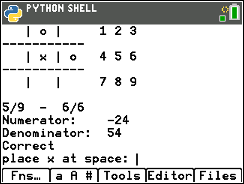•• ##### Device
•TI-84 Plus CE Python
• ##### Software

TI-SmartView™ CE

# Explorations with Coding: Fraction Tic Tac Toe

by Texas Instruments#### Objectives

• Use variables to store values
• Use the randint() function to generate integers
• Use the print() function to display
• Use a while loop to repeat code.
• Add and subtract fractions with unlike denominators.
• Multiply and divide fractions.(Teacher Note step 9 tells how to restrict to add and subtract)
• Add, subtract, multiple, and divide fractions with positive and negative values. (Teacher note step 3 to restrict to positive values).